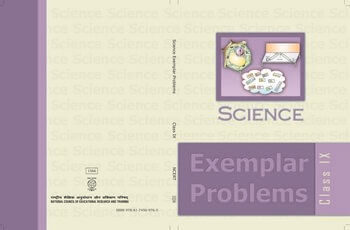•Long Answer Questions 34. A group of students took an old shoe box and covered it with a black paper from all sides. They fixed a source of light (a torch) at one end of the box by making a hole in it and made another hole on the other side to view the sample taken in a beaker/glass tumbler as shown in the figure. They were amazed to see that milk taken in the glass was illuminated. They tried the same activity by taking a salt solution but found that light simply passed through it (a) Explain why the milk sample was illuminated. Name the phenomenon involved. (b) Same results were not observed with a salt solution. Explain. (c) Can you suggest two more solutions which would show the same effect as shown by the milk solution? (2 + 2 +1 = 5) Or During an experiment the students were asked to prepare a 10% solution of sugar in water. Ramesh dissolved 10g of sugar in 100g of water while Sarika prepared it by dissolving 10g of sugar in water to make 100g of the solution. (a) Are the two solutions of the same concentration? (b) Compare the mass % of the two solutions. (1 + 4= 5) 35. Why do some children fall ill more frequently than others living in the same locality? (5) Or Give any four factors necessary for a healthy person. (5) 36. Define power. How will you differentiate between kW and kW h? If the velocity of a ball is tripled, what will be the ratio of (a) its initial kinetic energy and final kinetic energy (b) initial momentum and final momentum. (1 + 1 + 1½ + 1½ = 5) EXEMPLAR PROBLEMS